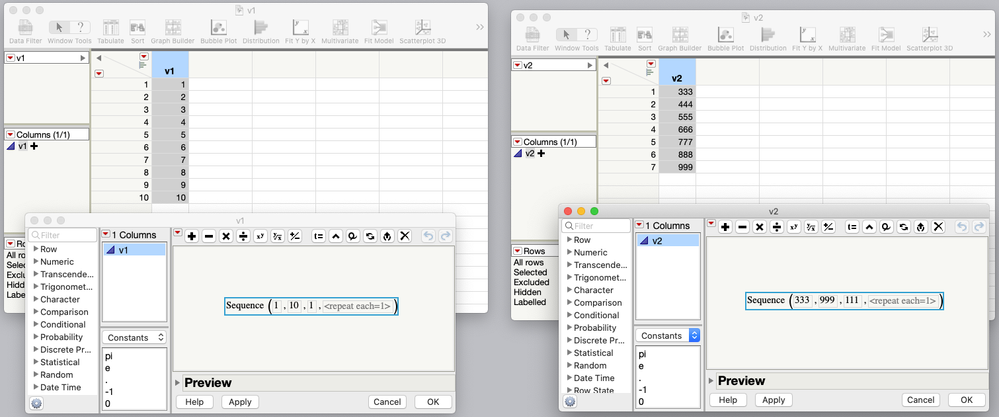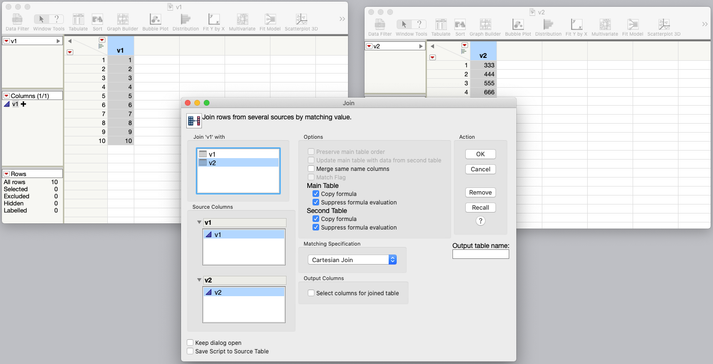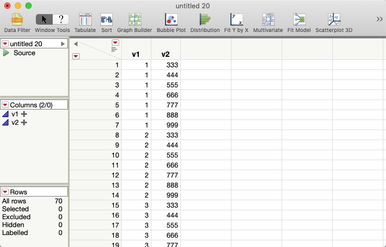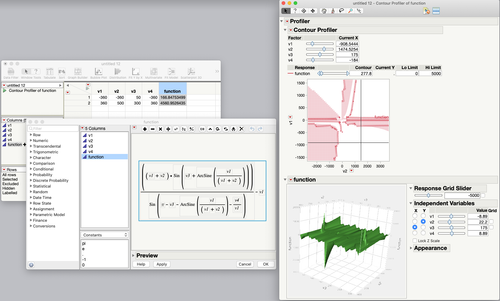Choose Language Hide Translation Bar
Highlighted

## Re: How do I plot mathematical functions with JMP?

Actually, Graph Builder is amazing for 4+ dimensions -- every drop-box in the UI is basically accepting a different dimension (though Map Shape and Interval might be exceptions). So here, I'd probably do y = y, x = v1, groupX = v2, groupY = v3, and overlay = v4. I've never even heard of the "Profiler", so I'll need to go learn about it. Thx!
Highlighted

## Re: How do I plot mathematical functions with JMP?

I did not mean to imply that Graph Builder is limited to just two variables. But visualizing this function would require some clear thinking about what you wanted to show and how you wanted to show it. I agree that GB can bring in more than two variables through different roles.

Also using the grouping and overlay roles limits the display to the levels in the column, so you would also have to build the data table more carefully to get the groups you want.

Learn it once, use it forever!
Highlighted

## Re: How do I plot mathematical functions with JMP?

I understand -- I think I was mostly interested in knowing *how* to build the data table if I knew what levels I wanted for each of the inputs. Basically, is there an easy way to build a full-factorial-matrix data table given a min, increment, and max value for each variable?
Highlighted

## Re: How do I plot mathematical functions with JMP?

Use the DOE > Classical > Full Factorial design platform. You can ignore or delete the meta-data that is created, too. You won't need it since you are not fitting empirical data.

Learn it once, use it forever!
Highlighted

## Re: How do I plot mathematical functions with JMP?

That platform seems limited to mostly single-digit number of values for each variable. I tried putting in "100" for the number of values, but the UI doesn't practically support that, the boxes to type in values are too small. Wish it would allow something like: 0:50:750
HighlightedJeff_PerkinsonCommunity Manager

## Re: How do I plot mathematical functions with JMP?

@BHarris wrote:
I understand -- I think I was mostly interested in knowing *how* to build the data table if I knew what levels I wanted for each of the inputs. Basically, is there an easy way to build a full-factorial-matrix data table given a min, increment, and max value for each variable?

Take a look at the Sequence() function (it's the Row functions in the Formula Editor),  and also Tables->Join, where you'll find a Cartesian join option.Two data tables, one column each, Sequence() formulaJoin Dialog, Cartesian Join optionResulting data table

-Jeff
Highlighted

## Re: How do I plot mathematical functions with JMP?

"Cartesian join", there it is. Thanks!
HighlightedJeff_PerkinsonCommunity Manager

## Re: How do I plot mathematical functions with JMP?

Following on @markbailey's suggestion, I'd look at the Contour Profiler as well.-Jeff
Article Labels